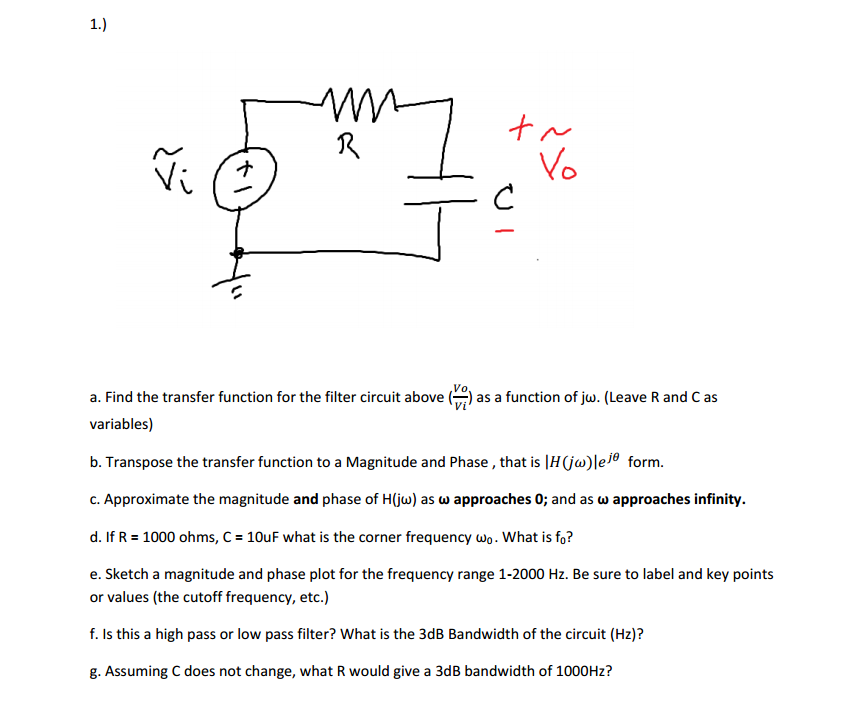Create an Account

Home / Questions / a Find the transfer function for the filter circuit above Vo Vi as a function of jw Leave ...

a Find the transfer function for the filter circuit above Vo Vi as a function of jw Leave R and C as variables b Transpose the transfer function to a magnitude and phase that is

a. Find the transfer function for the filter circuit above (Vo/Vi) as a function of jw. (Leave R and C as variables)

b. Transpose the transfer function to a magnitude and phase, that is |H(jw)|e^jtheta

c. Approximate the magnitude and phase, that is |H(jw)|e^jtheta

d. If R=1000 ohms, C=10uF what is the corner frequency w_o. What is f_o?

e. Sketch a magnitude and phase plot for the frequency range 1-2000 Hz. Be sure to label and key points or values (the cutoff frequency, etc.)

f. Is this a high pass or low pass filter? What is the 3dB Bandwidth of the circuit (Hz)?

g. Assuming C does not change, what R would give a 3dB bandwidth of 1000Hz?Show transcribed image text a. Find the transfer function for the filter circuit above (Vo/Vi) as a function of jw. (Leave R and C as variables) b. Transpose the transfer function to a magnitude and phase, that is |H(jw)|e^jtheta c. Approximate the magnitude and phase, that is |H(jw)|e^jtheta d. If R=1000 ohms, C=10uF what is the corner frequency w_o. What is f_o? e. Sketch a magnitude and phase plot for the frequency range 1-2000 Hz. Be sure to label and key points or values (the cutoff frequency, etc.) f. Is this a high pass or low pass filter? What is the 3dB Bandwidth of the circuit (Hz)? g. Assuming C does not change, what R would give a 3dB bandwidth of 1000Hz?

Jul 31 2020 View more View LessSubscribe To Get Solution